YOU CAN CODE!With The Case Of UCanCode.net  Release The Power OF  Visual C++ ! Home |  Products | Purchase |  Support | Downloads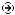Download EvaluationPricing & Purchase?E-XD++Visual C++/ MFC ProductsOverviewElectronic Form SolutionVisualization & HMI SolutionPower system HMI SolutionBar code labeling SolutionWorkflow SolutionCoal industry HMI SolutionInstrumentation Gauge SolutionReport Printing SolutionGraphical modeling SolutionGIS mapping solutionVisio graphics solutionIndustrial monitoring SolutionFlowchart and diagramming SolutionOrganization Diagram SolutionGraphic editor Source CodeUML drawing editor Source CodeMap Diagramming SolutionArchitectural Graphic Drawing SolutionRequest EvaluationActiveX COM ProductsOverviewTechnical Support# Get Ready to Unleash the Power of UCanCode .NET

UCanCode Software focuses on general application software development. We provide complete solution for developers. No matter you want to develop a simple database workflow application, or an large flow/diagram based system, our product will provide a complete solution for you. Our product had been used by hundreds of top companies around the world!

"100% source code provided! Free you from not daring to use components because of unable to master the key technology of components!"

 GDI Draw Curve Line: PolyBezier, PolyBezierTo

 Bézier Curves
 A bezier line is an arc that is strictly based on a set number of points instead of on an ellipse. A bézier curve uses at least four points to draw on. A bezier line with four points can be illustrated as follows: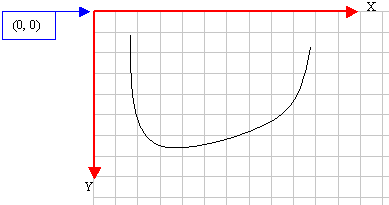To draw this line (with four points), the compiler would draw a curve from the first to the fourth points. Then it would bend the curve by bringing each middle (half-center) side close to the second and the third points respectively, of course without touching those second and third points. For example, the above bezier curve could have been drawn using the following four points: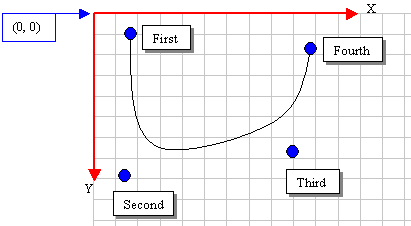PolyBezier(): To draw a bézier curve, the CDC provides the PolyBezier() method. Its syntax is:

`BOOL PolyBezier(const POINT* lpPoints, int nCount);`

The lpPoints argument is an array of POINT or CPoint values. The nCount argument specifies the number of points that will be used to draw the line. Here is an example:

 ```void CExoView::OnDraw(CDC* pDC) { CPoint Pt = { CPoint(20, 12), CPoint(88, 246), CPoint(364, 192), CPoint(250, 48) }; pDC->PolyBezier(Pt, 4); }```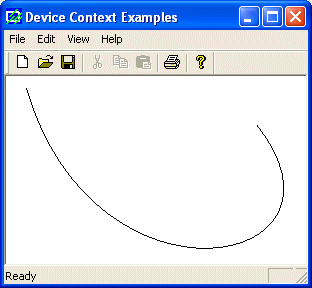In the same way, you can draw a series of complicated subsequent lines. This is done by adding reference points to the array. To do this, you must add points in increments of three. After drawing the first curve based on the first four points, to draw the next line, the function would use the fourth point as the starting point. Since the bezier line requires 4 points, you must add three more. You can continue adding points by three to draw the bezier curve. Here is an example:

 ```void CExoView::OnDraw(CDC* pDC) { CPoint Pt[] = {CPoint(20, 12), CPoint(88, 246), CPoint(364, 192), CPoint(250, 48), CPoint(175, 38), CPoint(388, 192), CPoint(145, 125) }; pDC->PolyBezier(Pt, 7); }```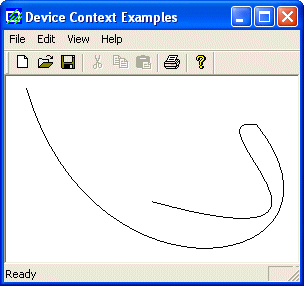PolyBezierTo(): The CDC::PolyBezier() method requires at least four points to draw its curve. This is because it needs to know where to start drawing. Another way you can control where the curve would start is by using the CDC::PolyBezierTo() method. Its syntax is:

`BOOL PolyBezierTo(const POINT* lpPoints, int nCount);`

The PolyBezierTo() method draws a bézier curve. Its first argument is a pointer to an array of POINT or CPoint values. This member function requires at least three points. It starts drawing from the current line to the third point. If you do not specify the current line, it would consider the origin (0, 0). The first and the second lines are used to control the curve. The nCount argument is the number of points that would be considered. Here is an example:

 ```void CExoView::OnDraw(CDC* pDC) { CPoint Pt[] = { CPoint(320, 120), CPoint(88, 246), CPoint(364, 122) }; pDC->PolyBezierTo(Pt, 3); }```